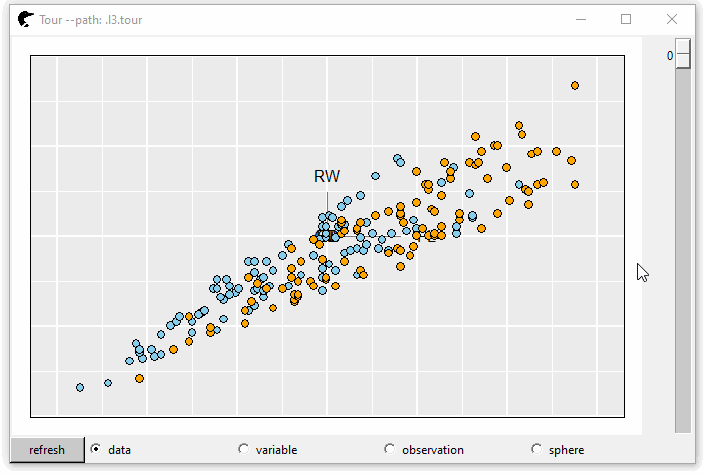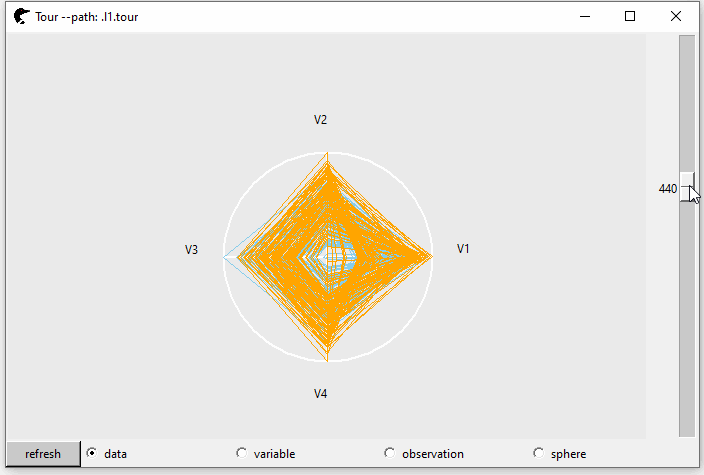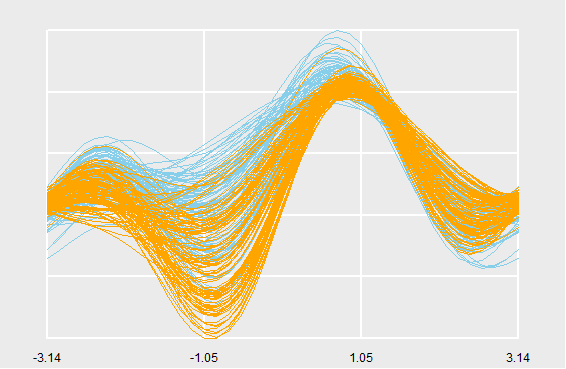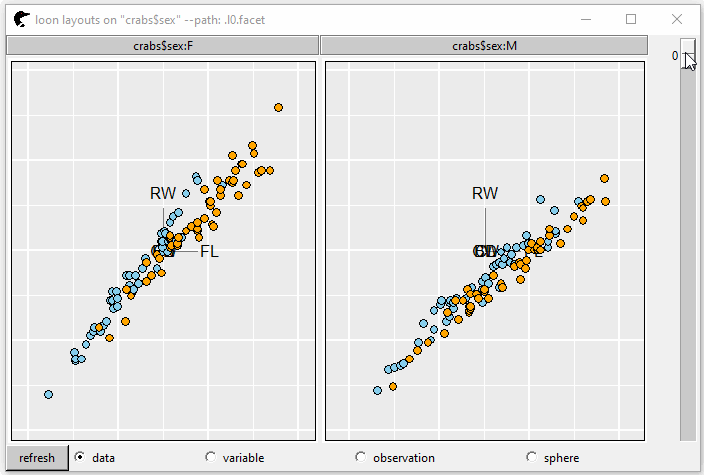# Tour in Loon

#### 2021-07-25

library(loon.tourr)

## Introduction

A tour is a motion graphic designed to study the joint distribution of multivariate data . A sequence of low-dimensional projections is created by a high dimensional data set. Tours are thus used to find interesting projections. Between each plane, interpolation along a geodesic path is provided so that the points in a sub space (e.g. 2D) can be rotated smoothly. In mathematics, $$\boldsymbol{X}_{n \times p}$$ represents the original data set; $$\boldsymbol{P}_{p \times d}$$ is the matrix of projection vectors where $$d < p$$. $\boldsymbol{Y} = \boldsymbol{X} \boldsymbol{P}$ where $$\boldsymbol{Y}$$ is the lower dimensional sub-space.

The tour was first implemented in the software Dataviewer in Symbolics Lisp machine. A smoothly moving scatterplot could be produced to visualize the tour paths. Then, implemented software XGobi in the X Window System, providing portability across a wide variety of workstations i.e. X terminals, personal computers, even across a network. Software GGobi , redesigned and extended its ancestor XGobi. It can be embedded in other software, like environment R. Package rggobi is an R interface of GGobi, however, it has been removed from the CRAN and can only be accessed from the archive.

Package tourr implements geodesic interpolation and variety tour generation functions (i.e. grand tour, guided tour, etc) in R language. The function animate provides tour animation as a kinematic sequence of static displays – plots are generated and then displayed quickly in order. In an RStudio display, for example, the user can cycle back and forth through the sequence … but that is all. Unlike earlier tour implementations (rggobi) no interactive manipulation of the plot elements is possible.

The loon package is a toolkit that enables highly interactive data visualization. The package loon.tourr adds the full functionality of loon’s interactive graphics to tourr. For example, this allows interactive selection, colouring, and deactivating of points in the tour display and linking that display with any other loon plot. Interesting projections discovered during the tour can be accessed at any point in the tour. In addition, random tours displaying more than 2 dimensions are also provided using parallel or radial coordinates and scatterplot matrices.

## Basics

The crabs data frame (stored in package MASS) contains 200 observations and 8 features, describing 5 morphological measurements on 50 crabs each of two colour forms and both sexes. GIF 1 shows the tours and color represents the species, “B” (blue) or “O” (orange).

library(MASS, quietly = TRUE)
kable(head(crabs, 6))
sp sex index FL RW CL CW BD
B M 1 8.1 6.7 16.1 19.0 7.0
B M 2 8.8 7.7 18.1 20.8 7.4
B M 3 9.2 7.8 19.0 22.4 7.7
B M 4 9.6 7.9 20.1 23.1 8.2
B M 5 9.8 8.0 20.3 23.0 8.2
B M 6 10.8 9.0 23.0 26.5 9.8

This plot is interactive. Users can pan, zoom or select on this plot. The default number of random bases is 30 and the steps between two serial projections are 40. So, we have 1200 + 1 (start position) projections in total. To navigate the tour, scroll the rightmost bar down, the projection is transformed from one to another. If, unfortunately, none of the projections is interesting, click the “refresh” button at the left-bottom corner. New random tours are created.

Immediately below the plot, behind the “refresh” button, there are several radio buttons, “data,” “variable,” “observation” and “sphere” that represent the scaling methods $$f$$ of $$\boldsymbol{X}$$.

• The first of which is “data,” where $$f(\boldsymbol{X}) = \boldsymbol{X}$$;

• If scaling = "variable", $$f(\mathbf{x}_j) = \frac{1}{a - b}(\mathbf{x}_j - b\mathbf{1})$$ where $$\boldsymbol{X} = [\mathbf{x}_{1}, ..., \mathbf{x}_{p}]$$, $$\mathbf{x}_{j}$$ is a $$n \times 1$$ vector, $$a = \max{(\mathbf{x}_j)}$$ and $$b = \min{(\mathbf{x}_j)}$$;

• The third is “observation,” where $$f(\mathbf{x}_i) = \frac{1}{c - d}(\mathbf{x}_i - d\mathbf{1})$$, $$\boldsymbol{X} = {[{\mathbf{x}_{1}}^{\mkern-1.5mu\mathsf{T}}, ..., {\mathbf{x}_{n}}^{\mkern-1.5mu\mathsf{T}}]}^{\mkern-1.5mu\mathsf{T}}$$, $$\mathbf{x}_{i}$$ is a $$p \times 1$$ vector, $$c = \max{(\mathbf{x}_i)}$$ and $$d = \min{(\mathbf{x}_i)}$$;

• If the scaling method is “sphere,” where $$f(\boldsymbol{X}) = \boldsymbol{X}^\star \boldsymbol{V}$$ where $$\boldsymbol{X}^\star = (\boldsymbol{I} - \frac{1}{n}\mathbf{1}{\mathbf{1}}^{\mkern-1.5mu\mathsf{T}})\boldsymbol{X} = \boldsymbol{U} \boldsymbol{D} \boldsymbol{V}$$.

Notice that the tour is based on the transformed data set $\boldsymbol{Y} = f(\boldsymbol{X}) \boldsymbol{P}$

color <- rep("skyblue", nrow(crabs))
color[crabs$sp == "O"] <- "orange" cr <- crabs[, c("FL", "RW", "CL", "CW", "BD")] p0 <- l_tour(cr, color = color)GIF 1: 2D grand tour The projection dimension (the dimension of the $$\boldsymbol{P}$$) is controlled by tour_path = grand_tour(d) where $$d$$ represents the dimensions and the default is $$2$$. Unlike the 2 dimensional Cartesian coordinate, higher dimensional space ($$>2$$) will be embedded in the parallel coordinate or radial coordinate. In GIF 2, the $$d$$ is set as 4. p1 <- l_tour(cr, tour_path = grand_tour(4L), color = color)GIF 2: 4D grand tour An l_tour object is returned by p1 (or p0). class(p1) # class(p0) # >  "l_tour" "loon"  The loon serialaxes plot for p1 (or scatterplot for p0) can be accessed by calling l_getPlots w <- l_getPlots(p1) class(w) # >  "l_serialaxes" "loon"  The matrix of projection vectors $$\boldsymbol{P}_{4 \times 4}$$ can be returned by function l_cget or a simple [ round(p1["projection"], 2) # > # [,1] [,2] [,3] [,4] # [1,] -0.12 -0.93 -0.01 -0.35 # [2,] -0.57 0.21 0.63 -0.36 # [3,] -0.06 0.14 0.14 -0.38 # [4,] -0.37 0.23 -0.76 -0.47 # [5,] -0.72 -0.14 -0.12 0.62 The radial coordinate can be converted to a parallel coordinate by calling [<- in the console or trigger the “parallel” radio button of “axes layout” on p1’s inspector. p1["axesLayout"] <- "parallel" Additionally, Andrews plot can be shown by runing the following code in console p1["andrews"] <- TRUE The interactive graphics can be turned to static either by plot(p1) or loon.ggplot::loon.ggplot(p1)Figure 1: Andrews curve To investigate more about loon, please visit great-northern-diver-loon. ## Tour techniques introduced several methods for choosing projections. In loon.tourr, by modifying the argument tour_path, all can be realized. • Grand tour: a sequence of projections is chosen randomly # Default, 2D grand tour pg <- l_tour(cr, tour_path = grand_tour(2L)) • Projection pursuit guided tour: a sequence of projections is guided by an algorithm in search of “interesting” projections by optimizing a criterion function $\mathop{\mathrm{arg\,max}}g(\boldsymbol{Y}), \forall \boldsymbol{P}$ where $$\boldsymbol{Y} = {[{\mathbf{y}}^{\mkern-1.5mu\mathsf{T}}_1, ..., {\mathbf{y}}^{\mkern-1.5mu\mathsf{T}}_n]}^{\mkern-1.5mu\mathsf{T}} = \boldsymbol{X} \boldsymbol{P}$$, $$\mathbf{y}_j$$ is a $$p \times 1$$ vector. • Holes: $g(\boldsymbol{Y}) = \frac{1 - \frac{1}{n}\sum_{i=1}^n \exp(-\frac{1}{2}{\mathbf{y}}^{\mkern-1.5mu\mathsf{T}}_i\mathbf{y}_i)}{1 - \exp{(-\frac{p}{2})}}$ # 2D holes projection pursuit indexes pp_holes <- l_tour(cr, tour_path = guided_tour(holes(), 2L)) • Central Mass: $g(\boldsymbol{Y}) = \frac{\frac{1}{n}\sum_{i=1}^n \exp(-\frac{1}{2}{\mathbf{y}}^{\mkern-1.5mu\mathsf{T}}_i \mathbf{y}_i) - \exp{(-\frac{p}{2})}}{1 - \exp{(-\frac{p}{2})}}$ # 2D CM projection pursuit indexes pp_CM <- l_tour(cr, tour_path = guided_tour(cmass(), 2L)) • LDA $g(\boldsymbol{Y}) = 1 - \frac{|{\boldsymbol{P}}^{\mkern-1.5mu\mathsf{T}}\boldsymbol{W}\boldsymbol{P}|}{|{\boldsymbol{P}}^{\mkern-1.5mu\mathsf{T}}(\boldsymbol{W} + \boldsymbol{B})\boldsymbol{P}|}$ where $$\boldsymbol{B} = \sum_{i=1}^k n_i (\bar{y}_{i.} - \bar{y}_{..}) {(\bar{y}_{i.} - \bar{y}_{..})}^{\mkern-1.5mu\mathsf{T}}$$, $$\boldsymbol{W} = \sum_{i=1}^k \sum_{j=1}^{n_i} n_i (\bar{y}_{ij} - \bar{y}_{i.}) {(\bar{y}_{ij} - \bar{y}_{i.})}^{\mkern-1.5mu\mathsf{T}}$$ and $$k$$ is the number of groups. # 2D LDA projection pursuit indexes pp_LDA <- l_tour(cr, color = crabs$sex,
tour_path = guided_tour(lda_pp(crabs$sex), 2L)) • Expect these, tourr also provides some other tour methods, for example: • frozen_tour, one variable is designated as the “manipulation variable,” and the projection coefficient for this variable is controlled; • little_tour, a planned tour that travels between all axis parallel projections; • local_tour, alternates between the starting position and a nearby random projection and etc. ## Compound plot ### Facets GIF 3 shows the tour plot separated by variable sex into two panels. pf <- l_tour(cr, by = crabs$sex,
color = color)GIF 3: facets

### Pairs plot

GIF 4 shows the tour pairs plot (the upper triangle). If we set showSerialAxes = TRUE, a serial axes plot (parallel or radial axes) is displayed at the lower triangle.

pp <- l_tour_pairs(cr,
tour_path = grand_tour(4L),
color = color,
showSerialAxes = TRUE)